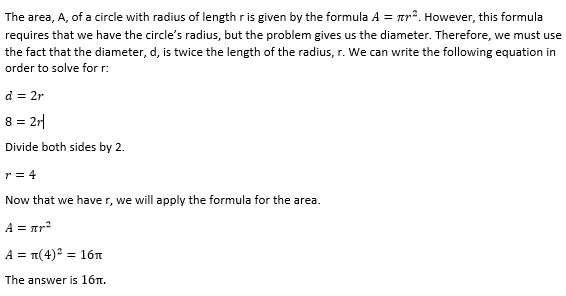Example Questions

Example Question #82 : Geometry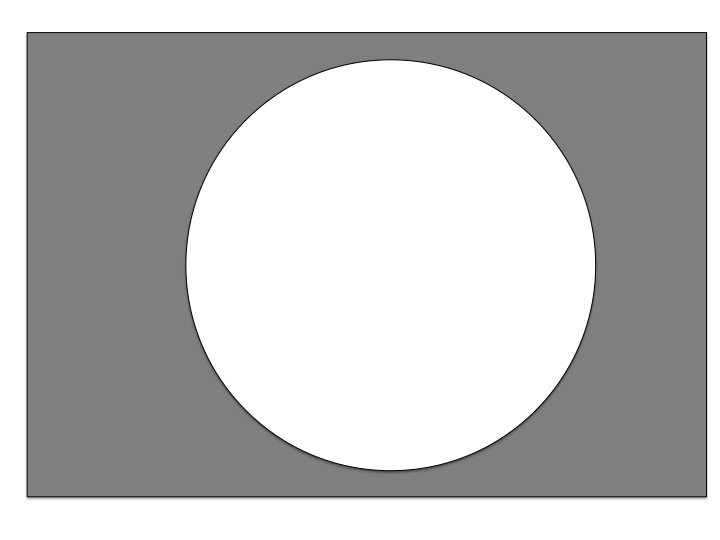The picture above contains both a circle with diameter 4, and a rectangle with length 8 and width 5. Find the area of the shaded region. Round your answer to the nearest integer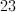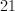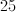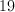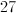Explanation:

First, recall that the diameter of a circle is twice the value of the radius. Therefore a circle with diameter 4 has a radius of 2. Next recall that the area of a circle with radius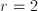is: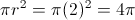The area of the rectangle is the length times the width: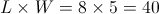The area of the shaded region is the difference between the 2 areas: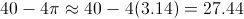The nearest integer is 27.

Example Question #83 : Geometry

Allen was running around the park when he lost his keys.  He was running around aimlessly for the past 30 minutes.  When he checked 10 minutes ago, he still had his keys.  Allen guesses that he has been running at about 3m/s.

If Allen can check 1 square kilometer per hour, what is the longest it will take him to find his keys?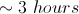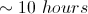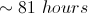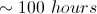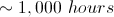Explanation:

Allen has been running for 10 minutes since he lost his keys at 3m/s.  This gives us a maximum distance of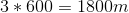from his current location.  If we move 1800m in all directions, this gives us a circle with radius of 1800m.  The area of this circle is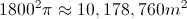Our answer, however, is asked for in kilometers.  1800m=1.8km, so our actual area will be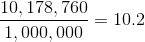square kilometers.  Since he can search 1 per hour, it will take him at most 10.2 hours to find his keys.

Example Question #21 : Basic Geometry

A 12x16 rectangle is inscribed in a circle. What is the area of the circle?

120π

90π

100π

50π

10π

100π

Explanation:

Explanation: Visualizing the rectangle inside the circle (corners touching the circumference of the circle and the center of the rectangle is the center of the circle) you will see that the rectangle can be divided into 8 congruent right triangles, with the hypotenuse as the radius of the circle. Calculating the radius you divide each side of the rectangle by two for the sides of each right triangle (giving 6 and 8). The hypotenuse (by pythagorean theorem or just knowing right triangle sets) the hypotenuse is give as 10. Area of a circle is given by πr2. 102 is 100, so 100π is the area.

A circle is inscribed in a square whose side is 6 in. What is the difference in area between the square and the circle, rounded to the nearest square inch?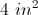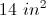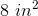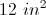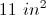Explanation:

The circle is inscribed in a square when it is drawn within the square so as to touch in as many places as possible. This means that the side of the square is the same as the diameter of the circle.

Let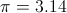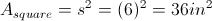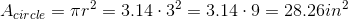So the approximate difference is in area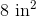Example Question #101 : Geometry

Two equal circles are cut out of a rectangular sheet of paper with the dimensions 10 by 20. The circles were made to have the greatest possible diameter. What is the approximate area of the paper after the two circles have been cut out?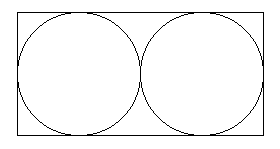56

16

43

23

43

Explanation:

The length of 20 represents the diameters of both circles. Each circle has a diameter of 10 and since radius is half of the diameter, each circle has a radius of 5. The area of a circle is A = πr2 . The area of one circle is 25π. The area of both circles is 50π. The area of the rectangle is (10)(20) = 200. 200 - 50π gives you the area of the paper after the two circles have been cut out. π is about 3.14, so 200 – 50(3.14) = 43.

Example Question #102 : Geometry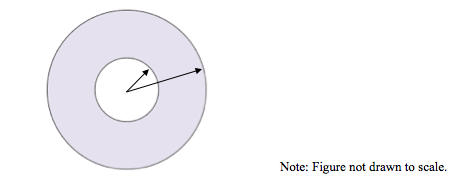Kate has a ring-shaped lawn which has an inner radius of 10 feet and an outer radius 25 feet. What is the area of her lawn?

175π ft2

275π ft2

325π ft2

525π ft2

125π ft2

525π ft2

Explanation:

The area of an annulus is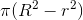whereis the radius of the larger circle, andis the radius of the smaller circle.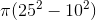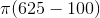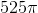Example Question #103 : Geometry

A 6 by 8 rectangle is inscribed in a circle. What is the area of the circle?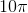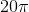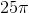Explanation:

The image below shows the rectangle inscribed in the circle. Dividing the rectangle into two triangles allows us to find the diameter of the circle, which is equal to the length of the line we drew. Using a2+b2= c2 we get 6+ 82 = c2. c2 = 100, so c = 10. The area of a circle is. Radius is half of the diameter of the circle (which we know is 10), so r = 5.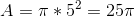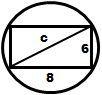Example Question #104 : Geometry

A park wants to build a circular fountain with a walkway around it.  The fountain will have a radius of 40 feet, and the walkway is to be 4 feet wide.  If the walkway is to be poured at a depth of 1.5 feet, how many cubic feet of concrete must be mixed to make the walkway?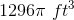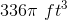None of the other answers are correct.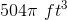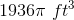Explanation:

The following diagram will help to explain the solution: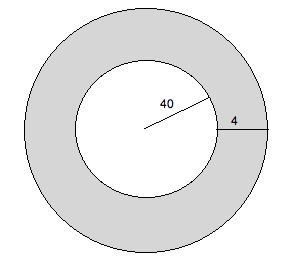We are searching for the surface area of the shaded region.  We can multiply this by the depth (1.5 feet) to find the total volume of this area.

The radius of the outer circle is 44 feet.  Therefore its area is 442π = 1936π.  The area of the inner circle is 402π = 1600π.  Therefore the area of the shaded area is 1936π – 1600π = 336π.  The volume is 1.5 times this, or 504π.

Example Question #7 : Area Of A Circle

How many times greater is the area of a circle with a radius of 4in., compared to a circle with a radius of 2in.?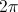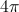Explanation:

The area of a circle can be solved using the equation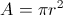The area of a circle with radius 4 is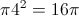while the area of a circle with radius 2 is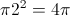.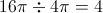Example Question #105 : Geometry

What is the area of a circle whose diameter is 8?

8π

12π

32π

64π

16π